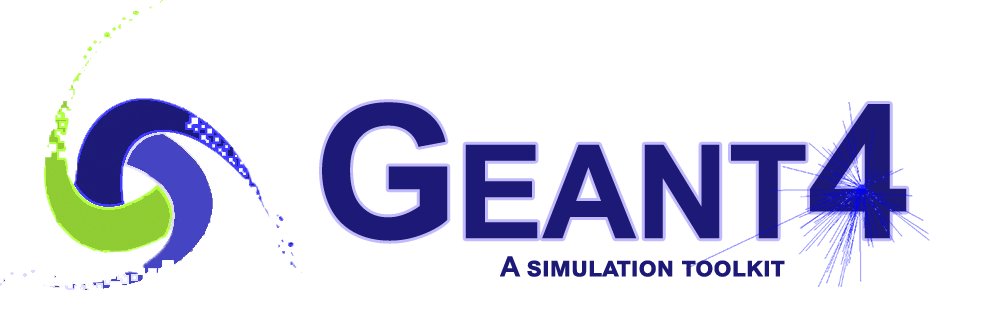How to use if conditions in geant4, restricting it to a certain volume of the world?

Let’s suppose i want that some information, maybe electric field, maybe magnetic field or other field, be restricted only to a certain region of the world volume. How could i achieve this?

I have read a code that is like this:

if (20.003*mm >= sqrt(pow(Point*mm,2)+pow(Point*mm,2))) {
.... //where ... here would contain the information to be restricted only at the positions above.
}

So, is that the right way to restrict a information under some region of the space? In this case, a disk. What does it means Point or Point?
Could it be something like that?

if (20.003*mm >= sqrt(pow(x,2)+pow(y,2))) {
.... //where ... here would contain the information to be restricted only at the positions above.
}

Generally I do that in post-processing/analysis of the file generated by G4. In your case, may be you can implement that in SteppingAction.cc and use step->GetPreStepPoint() or step->GetPostStepPoint() and restrict the fetched point (x,y,z) to the physical domain of your interest?

See following URLs:

G4bool Mensdec::ProcessHits(G4Step *aStep, G4TouchableHistory *ROhist)
{
G4Track *track = aStep->GetTrack();
G4StepPoint *preStepPoint = aStep->GetPreStepPoint();
G4ThreeVector particle = preStepPoint->GetPosition();
float x = particle*mm;
float y = particle*mm;
float z = particle*mm;
}

void Campo::GetFieldValue(const G4double Point, G4double field) const{

field=0.*tesla;
field=0.*tesla;
field=0.*tesla;
field=0.*volt/mm;

if (20.003*mm >= sqrt(pow(x,2)+pow(y,2))) {
field=0;
field=0;
}
else if (sqrt(pow(x,2)+pow(y,2))>20.003*mm and 91.003*mm>=sqrt(pow(x,2)+pow(y,2))) {
auto V = -2530*volt;
auto a = (1800*volt+V)/(log(20.003/91.003));
field=a*(x/(pow(y,2)+pow(x,2)));
field=a*(y/(pow(y,2)+pow(x,2)));
}
else if(sqrt(pow(x,2)+pow(y,2))>91.003*mm){
auto V = -2530*volt;
auto b = -(2550*volt+V)/(log(91.003/96.003));
field=b*(x/(pow(y,2)+pow(x,2)));
field=b*(y/(pow(y,2)+pow(x,2)));
}
}

Thank you.
I have writed this. Do you think is it right? The compiler run and the visualization open as well.